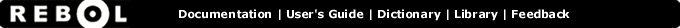# Strict-equal? - Function Summary

## Summary:

Returns TRUE if the values are equal and of the same datatype.

## Usage:

strict-equal? value1 value2

## Arguments:

value1 - The value1 argument.

value2 - The value2 argument.

## Description:

Strict equality requires the values to not differ by datatype (so 1 would not be equal to 1.0) nor by character casing (so "abc" would not be equal to "ABC").

 ``` print strict-equal? 123 123 true```

## Related:

<> - Returns TRUE if the values are not equal.
= - Returns TRUE if the values are equal.
== - Returns TRUE if the values are equal and of the same datatype.